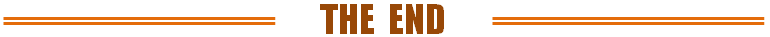图像的同态滤波原理及实现================================================================================

f = log(1+I);                          % log transformation

fFFT，此步涉及后续的频域滤波。因此，FFT前数据f一般需零填充至本身的2倍尺寸。

Fp = fft2(f,P,Q);                   % FFT with zeros padding

[v, u] = meshgrid(1:Q, 1:P);  % [y, x] = size(I)，y为行数，x为列数。与习惯顺序[x, y]有点反，易混淆！

u = u - floor(P/2);                  % u方向 centralization

v = v - floor(Q/2);                  % v方向 centralization

D = u.^2 + v.^2;                      % compute the distances

H = 1-exp(-c*(D./D0^2));    % gaussian high-pass filter

H = (rH - rL)*H + rL;           % homomorphic filter

H   = ifftshift(H);          % H反中心化，因Fp未中心化！

Gp = Fp.*H;                   % filtering in frequency domain

gp  = real(ifft2(Gp));    % IFFT取实部。网上有些代码直接取abs，计算误差会引起虚部不为零。No!

g    = gp(1:M,1:N);        % 截取有效数据

g = exp(g)-1;                % 先前取对数时，为log(1+I)。 有代码先在频域做exp(Gp)，再反变换。No!《教学后记》序列博文：

https://wap.sciencenet.cn/blog-425437-1052070.html

全部精选博文导读

GMT+8, 2022-1-25 10:28